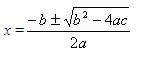## Mar 11, 2010

### DiscriminantThe term which is inside the square root symbol is called the discriminant.
It is used to determine the nature of the roots of a quadratic equation. We can also determine the number of real roots for a quadratic equation with this number. The following table will give us the relation between the discriminant and the nature of the roots.
 Discriminant Nature of roots Number of real roots b2 - 4ac > 0 Two real roots 2 b2 - 4ac = 0 Double root 1 b2 - 4ac < 0 No real roots 0
Example 1:
Find the discriminant value for the equation x2 + 5x + 6 = 0 and determine the number of real roots.
Solution:
Step 1:
Given equation is x2 + 5x + 6 = 0.
It is in the form ax2 + bx + c = 0, where a = 1, b = 5, and c = 6.
Step 2:
The discriminant = b2 - 4ac
b2 - 4ac = (5)2 - 4(1)(6) = 25 - 24 = 1 > 0
=> The equation has two real roots.
=> The graph of this equation will touch the x-axis twice.
Step 3:
So, the given equation has two real roots.
Example: 2
Find the discriminant value of x2 - 12x + 36 = 0 and determine the number of real roots.
Solution:
Step 1:
Given equation is x2 - 12x + 36 = 0.
It is in the form ax2 + bx + c = 0 where, a = 1, b = -12, and c = 36.
Step 2:
Discriminant = b2 - 4ac = (-12)2 - 4(1)(36) = 144 - 144 = 0
=> The equation has a double root.
=> The graph of this equation touches the x-axis in only one point.
Step 3:
So, the given equation has a double root.
Example: 3
Find the discriminant value 2x2 + x + 3 = 0 and determine the number of real roots.
Solution:
Step 1:
The given quadratic equation is 2x2 + x + 3 = 0.
It is in the form ax2 + bx + c = 0 where, a = 2, b = 1, and c = 3.
Step 2:
b2 - 4ac = (1)2 - 4(2)(3) = 1 - 24 = -23 < 0
=> The equation has no real roots.
=> The graph of this equation does not touch the x-axis.
Step 3:
So, the given quadratic equation has no real roots.
Related Articles: